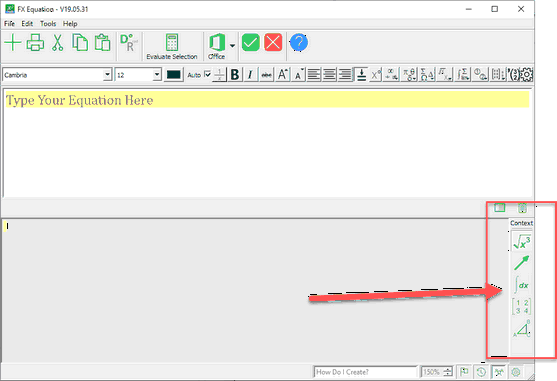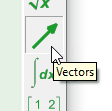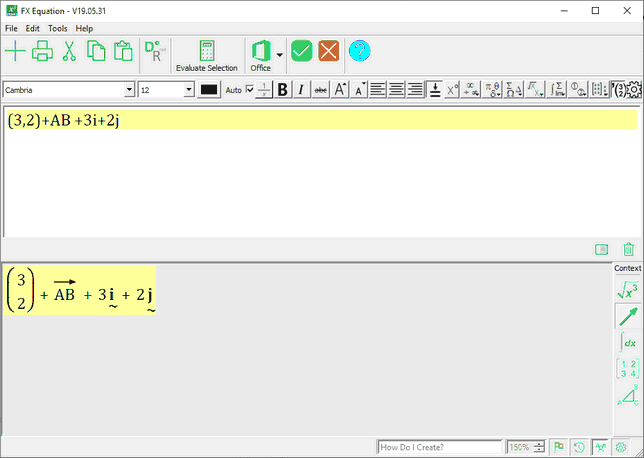﻿ Equation Contexts

# Equation Contexts

Top  Previous  Next

In May 2019, we added a new concept to FX Equation - equation contexts.# What Are Equation Contexts?

Equation contexts are a quick way to tell FX Equation the type of equation you are working on at the moment. This allows FX Equation to set up its options to suit that type of equation. For example, choosing the Vector context...sets up FX Equation so that typing commas produces column vectors; typing double capitals adds an arrow above and FX Equation will take its best guess at lower case vectors.There are five contexts available:

 • General Algebra (used for most equations).
 • Vectors.
 • Calculus.
 • Matrices.
 • Geometry.
 • Sets.